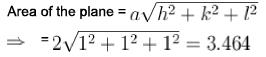Courses

# Crystals NAT Level – 1

## 10 Questions MCQ Test Topic wise Tests for IIT JAM Physics | Crystals NAT Level – 1

Description
This mock test of Crystals NAT Level – 1 for Physics helps you for every Physics entrance exam. This contains 10 Multiple Choice Questions for Physics Crystals NAT Level – 1 (mcq) to study with solutions a complete question bank. The solved questions answers in this Crystals NAT Level – 1 quiz give you a good mix of easy questions and tough questions. Physics students definitely take this Crystals NAT Level – 1 exercise for a better result in the exam. You can find other Crystals NAT Level – 1 extra questions, long questions & short questions for Physics on EduRev as well by searching above.
*Answer can only contain numeric values
QUESTION: 1

### A crystal belongs to a face cubic lattice with four atoms in the unit cell. The size of crystal is 1cm and its unit cell dimension is 1nm.Then the number of atoms in the crystal is in unit of 1021  is?

Solution:

Crystal size = 1 cm
Size of unit cell = 1 nm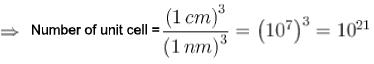For fee structure, number of atoms per unit cell = 4
Number of atoms = 4x1021

*Answer can only contain numeric values
QUESTION: 2

### Metallic monovalent sodium crystallizes in bcc structures. If the length of unit cell is 4 x 10-8 cm. The concentration of conduction electrons in metallic sodium is x * 1022 cm-3 . Find x?

Solution:

Sodium has one conduction electron and bcc structure, for bcc structure, number of atoms in unit cell = 2.
Number of conduction electrons = 2x1 =2.
Concentration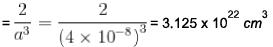*Answer can only contain numeric values
QUESTION: 3

### Atomic radius of copper (fcc) is 1.28Â , then density of copper is (kg/m3 )

Solution: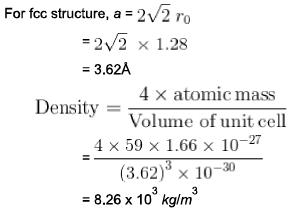*Answer can only contain numeric values
QUESTION: 4

Nearest neighbour distance in Na crystal is 1 . 8 3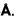Density of electrons in Na crystal in m-3 is :

Solution:

Na has bcc structure.
Nearest neighbour distance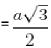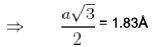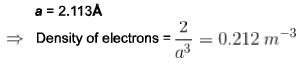*Answer can only contain numeric values
QUESTION: 5

Molecular weight of KCI (fee) is 74.6amu and its density is 1.99 x 103 kg/m3 . The lattice constant of KCI in units of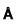is :

Solution: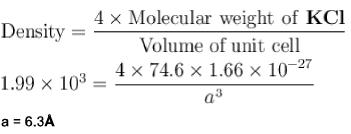*Answer can only contain numeric values
QUESTION: 6

Smallest angle of diffraction for an X-ray beam of wavelength 0.71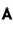is (in degrees).

Solution:

For [1 1 1] Plane,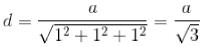For smallest angle,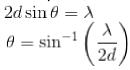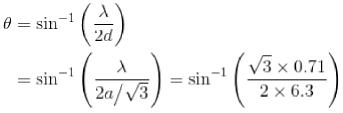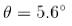*Answer can only contain numeric values
QUESTION: 7

The value of lattice constant a for a fee having in density 650 kg/m3 and atomic weight 60.2 in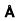is :

Solution:

Lattice constant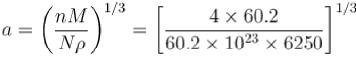a = 4.

*Answer can only contain numeric values
QUESTION: 8

If the angle between direction of X-ray (incident) and diffracted one is 16°, the angle of incidence in degrees will be :

Solution: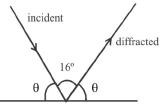⇒ 2θ = 180°
⇒ θ = 82°

*Answer can only contain numeric values
QUESTION: 9

If 0.28nm is spacing between the nearest neighbouring ions in NaCI lattice, the unit cell parameter in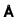is?

Solution:

r - 0.28 nm
⇒ 2r = 0.56 nm
= 5.6*Answer can only contain numeric values
QUESTION: 10

If the miller indices for a plane is [1 1 1] and 2 be the lattice constant, then what is the area of the plane ?

Solution: